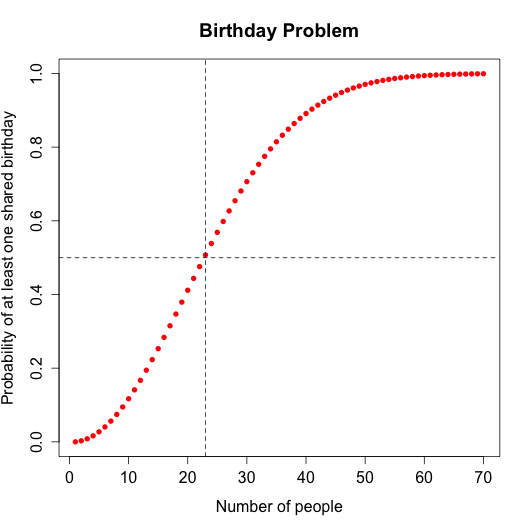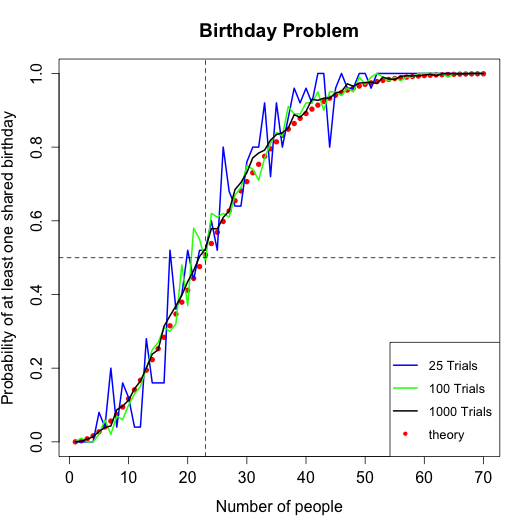# Bday-Problem-in-R

Created: 2014-05-19 16:18
Updated: 2016-10-15 06:16

#Birthday problem simulation in R This is a project in which I run a simulation of the birthday problem.

##Brithday problem

What is the probability that in a set of n randomly chosen people at least two will have the same birthday?

According to theory, the probability will be approximately 1 for a group of sixty people. A formula used to calculate the theoretical probabilities iswhere p(n) is the probability that at least two people share the same birthday in a group of n people. Below is the graph of theoritical probabilities against the number of people in a group.The horizontal dashed line is drawn at a point 0.5 and the vertical dashed line is drawn at a point 23. In other words, the theoretical probability of a shared birthday in a group of 23 people is approximately 0.5 or 50%.

I calculated the theoretical probabilities in Mathematica. The Mathematica notebook and the csv file with theoritical probabilities are in the `Mathematica` folder.

##Simulation I run the simulation and analysis in `bdayProblem.R` script. The function `bdayProbs()` is the actual simulation. It takes two arguments:

• number of people
• number of trials

For example, `bdayProbs(60,25)` will return a dataframe of probabilities of a shared birthday in group of all sizes up to 60 people. The group of each size will be drawn 25 times. The function will record each time a group had a shared birthday, and then divide the number of times a group had a shared birthday by the number of trials that is by 25. In other words, we get a fraction of times the group had a shared birthday which is in essence the probability of a shared birthday in a group.

The simulated probabilites for 25, 100, and 1000 trials as well as theoretical probabilities are in a graph below.We can see that the simulated probabilities approach the theorietical probabilties as the number of trials is increasing. Therefore, theory is backed up by the simulated data. So, in a class of sixty people (probably a lecture) there is 99.9% probability that at least two people share a birthday.